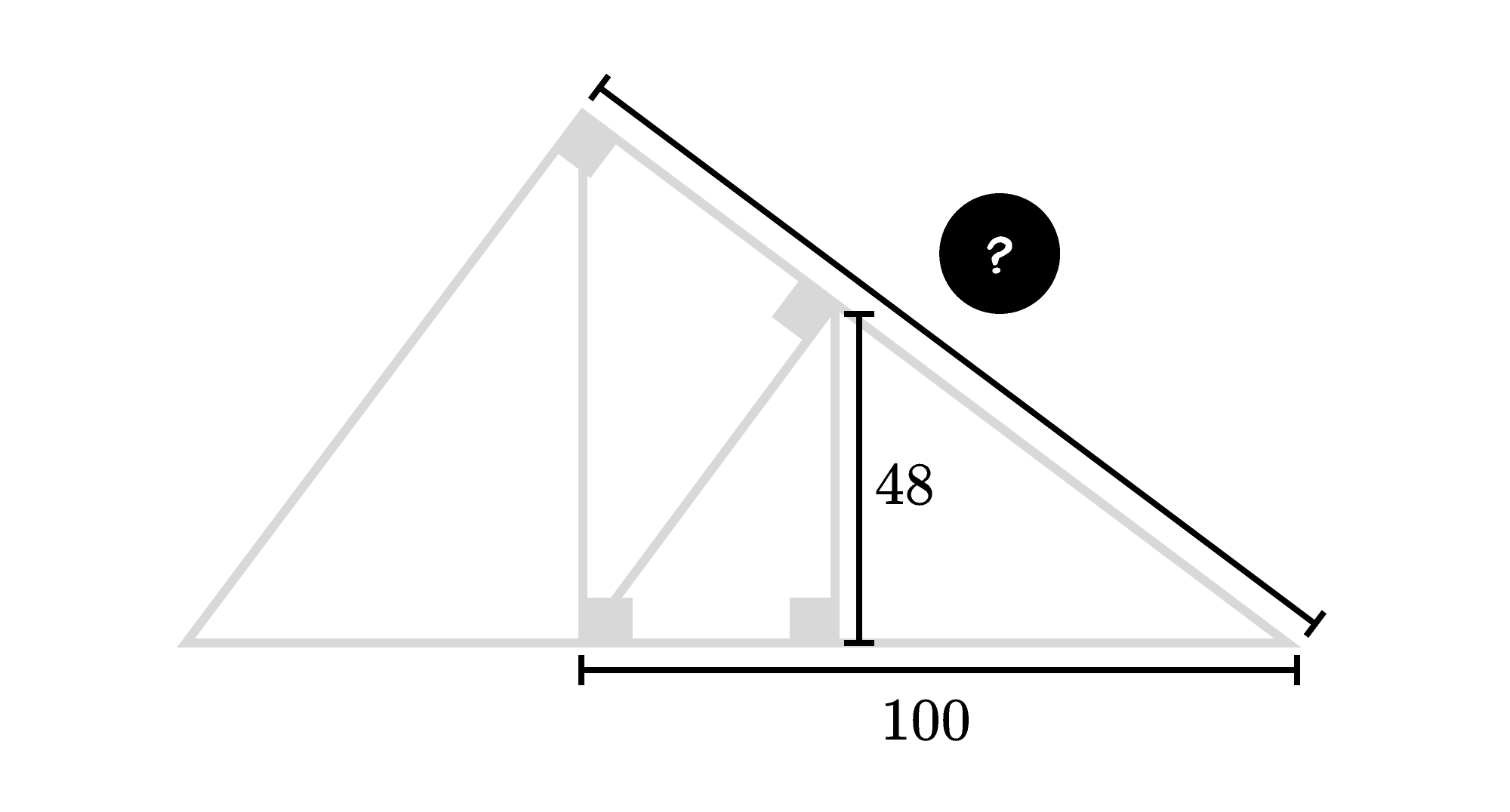## A Triangle Nest

How can we find the missing side lengths of the large right triangle?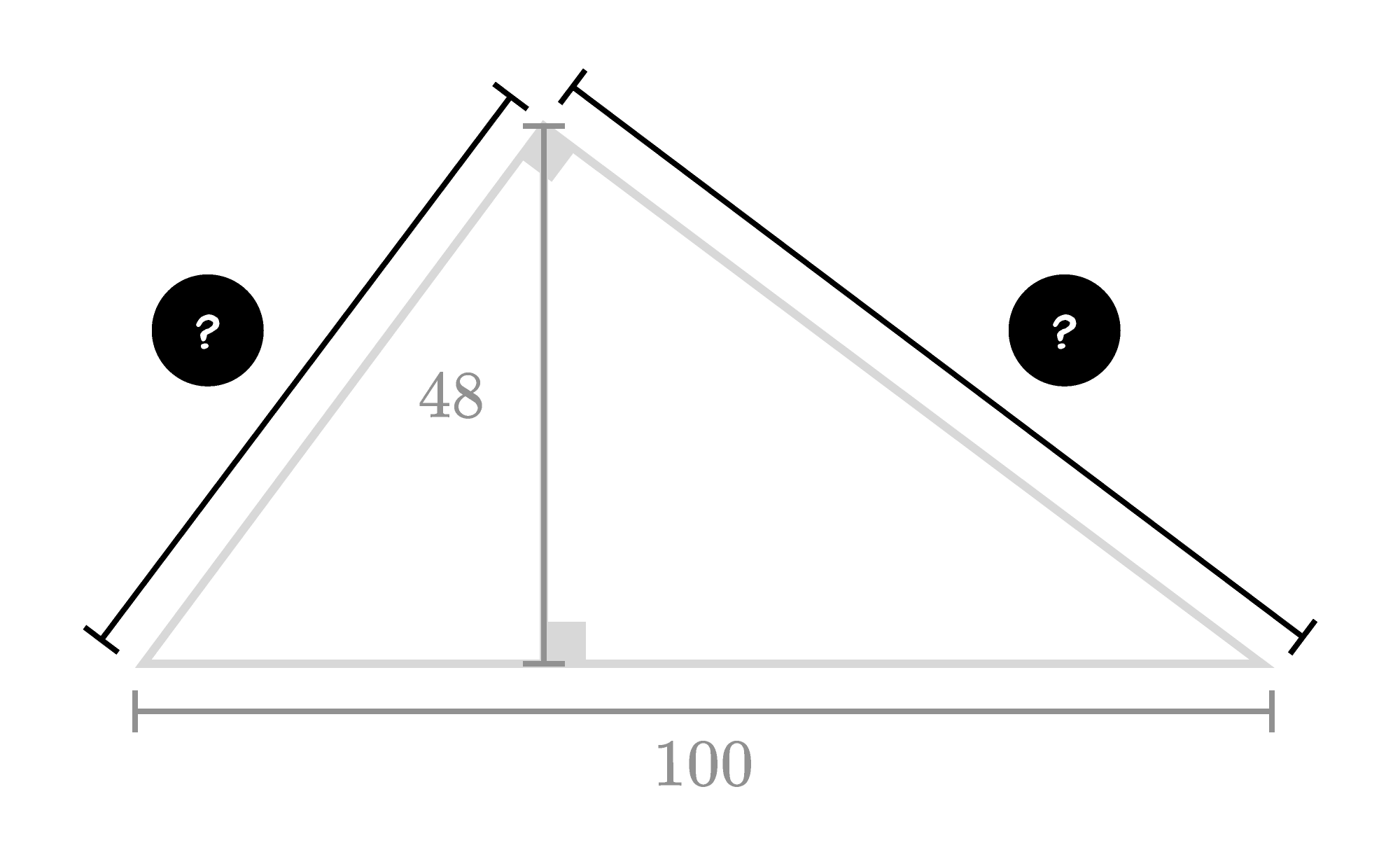Keep reading to see one way of breaking things down to find these lengths. If you feel ready for a more extended problem, jump ahead to the challenge.

Sometimes it's best to start by breaking a problem down into pieces and then seeing how the pieces are related. To do that here, we're going to need to introduce some variables. The segment with length $48$ splits the right triangle into two smaller right triangles. Two of their side lengths add up to $100;$ let's call them $a$ and $b.$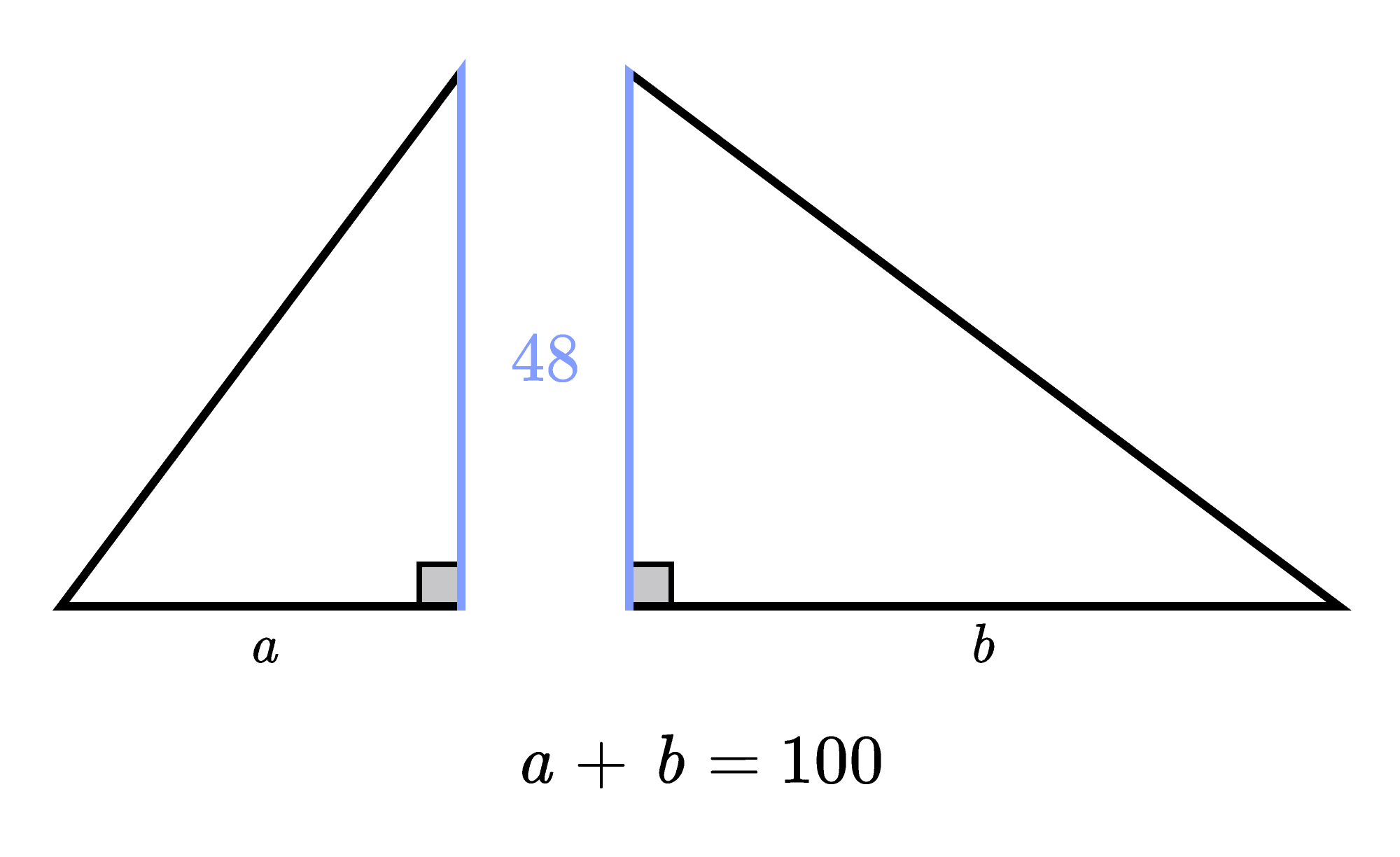That still doesn't tell us very much about how these two triangles are related. We looked at some of their sides; let's now look at their angles. Every triangle's measures add up to $180^\circ.$ Since each of these triangles has a $90^\circ$ angle, its two acute angles' measures add up to $90^\circ.$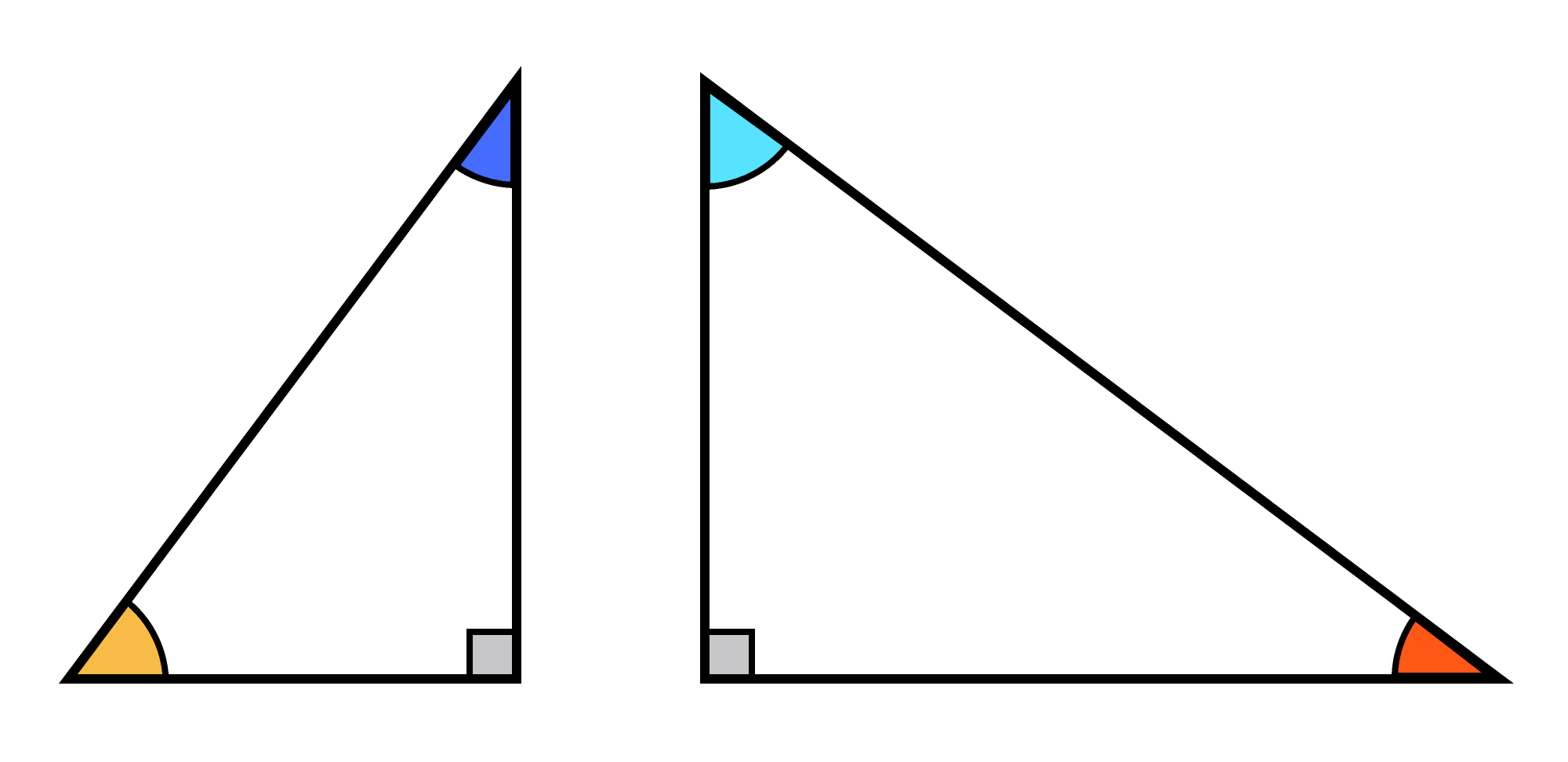But then the upper two acute angles of the two triangles form a right angle, so their measures also add up to $90^\circ.$ What does this mean?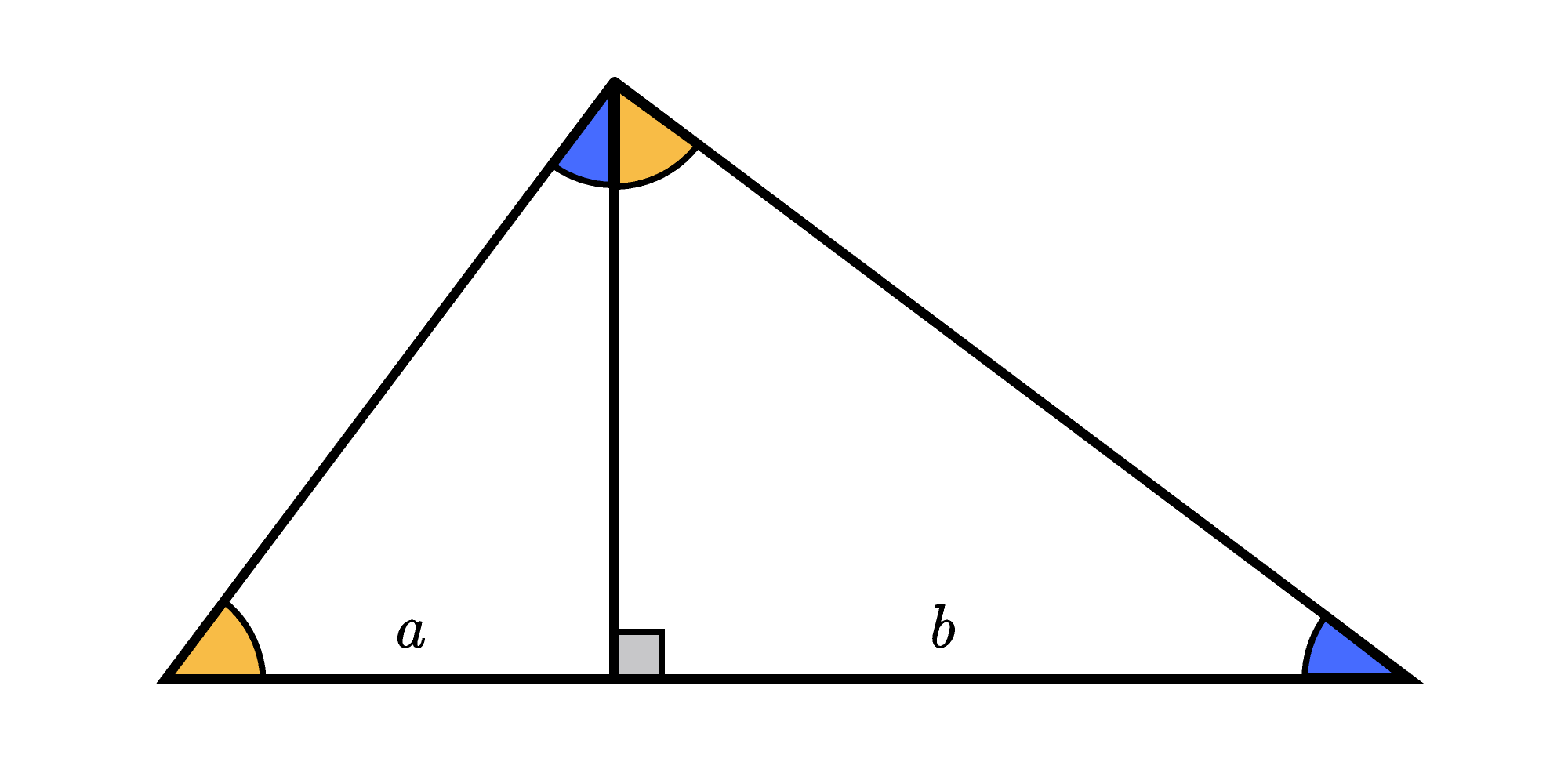The two triangles have congruent angles, so they are similar. This happens every time you split a right triangle with an altitude through its right angle.

We already knew that two of the triangles' side lengths add up to $100.$ Now that we know the triangles' side lengths are proportional, we have another way of relating them:

$\frac{a}{48} = \frac{48}{b} \implies ab = 48^2.$

We need two numbers with a product of $48^2$ and a sum of $100.$ Let's think about prime factors to help to guess and check our way to a solution. (This will only help if the triangle's sides are integers, which we don't know for sure at this point, but we can hope…)

Since $48 = 2^4 \times 3,$ we know that $48^2 = 2^8 \times 3^2.$ If $a$ and $b$ are integers, they must be made of these prime factors. For example, $a = 2^3 \times 3 = 24$ and $b = 2^5 \times 3 = 96$ have a product of $2^8 \times 3^2.$ Their sum, though, is $24 + 96 = 120,$ which is too large.

The combination of factors that does work is $a = 2^6 = 64$ and $b = 2^2 \times 3^2 = 36,$ since $36 + 64 = 100.$Finally, we can use the Pythagorean theorem to get the missing side lengths:

\begin{aligned} 64^2 + 48^2 & = 6400 \\ \sqrt{6400} & = 80 \\\\ 36^2 + 48^2 & = 3600 \\ \sqrt{3600} & = 60. \end{aligned}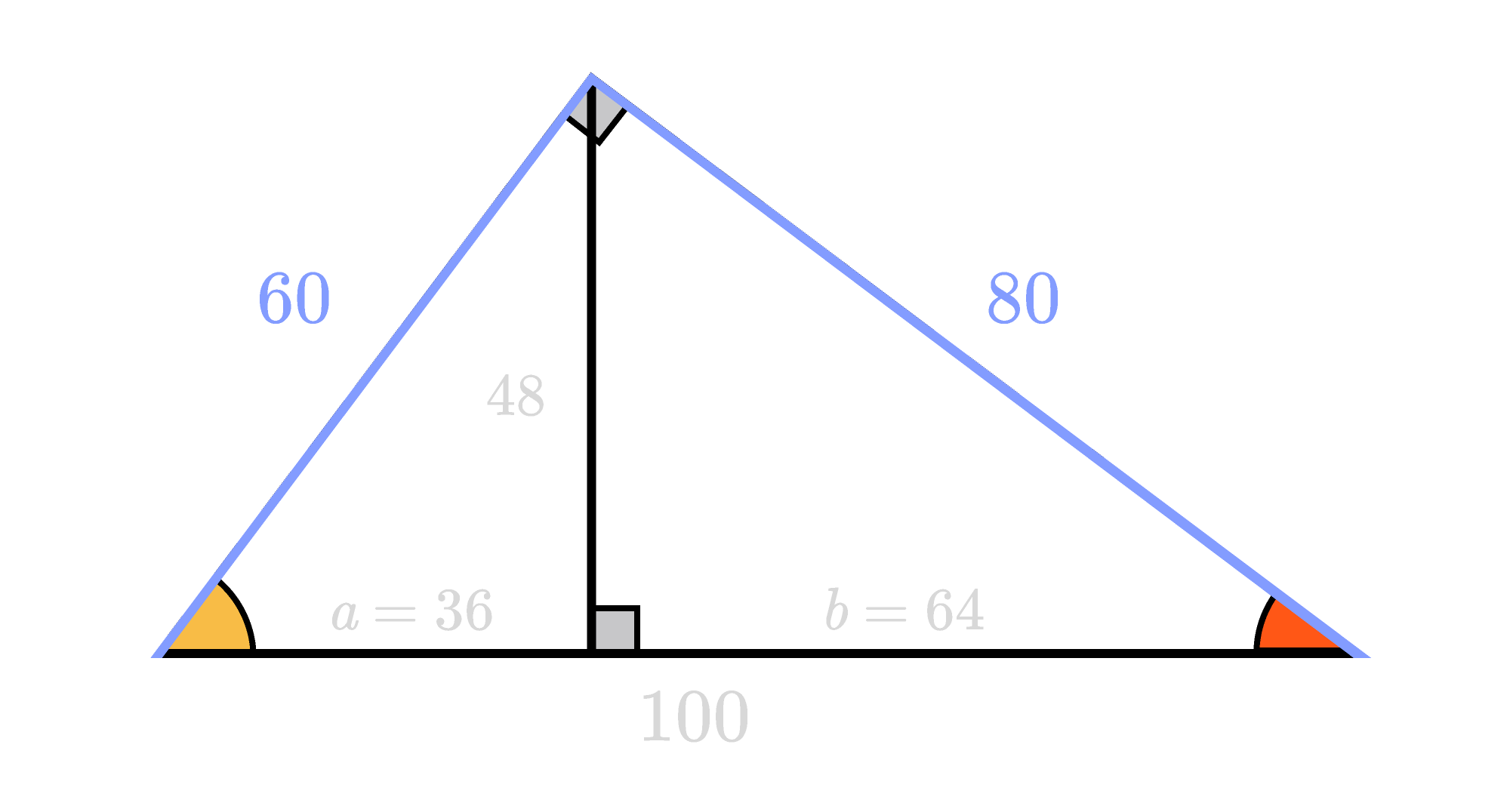Can you take this a step further to solve the challenge below?

# Today's Challenge

What is the missing length?